# 先介绍一下基本概念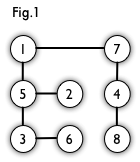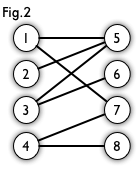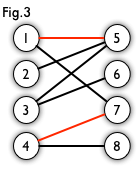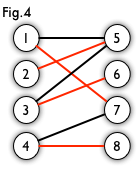# 代码如下：

#include<bits/stdc++.h>
#define MAXN 9999
using namespace std;
int nx,ny;//nx表示二分图左边顶点的个数，ny表示二分图右边顶点的个数
int m;//m代表边的条数
int cx[MAXN],cy[MAXN];//如果有cx[i]=j，则必有cy[j]=i，说明i点和j点能够匹配
int x,y;//x点到y点有边
int e[MAXN][MAXN];//邻接矩阵
int visited[MAXN];//标记数组，标记的永远是二分图右边的顶点
int ret;//最后结果
int point(int u)//这个函数的作用是寻找增广路和更新cx，xy数组，如果找到了增广路，函数返回1，找不到，函数返回0。
{
for(int v=1;v<=ny;v++)//依次遍历右边的所有顶点
{
if(e[u][v]&&!visited[v])//条件一：左边的u顶点和右边的v顶点有连通边，条件二：右边的v顶点在没有被访问过，这两个条件必须同时满足
{
visited[v]=1;//将v顶点标记为访问过的
if(cy[v]==-1||point(cy[v]))//条件一：右边的v顶点没有左边对应的匹配的点，条件二：以v顶点在左边的匹配点为起点能够找到一条增广路（如果能够到达条件二，说明v顶点在左边一定有对应的匹配点）。
{
cx[u]=v;//更新cx，cy数组
cy[v]=u;
return 1;
}
}
}
return 0;//如果程序到达了这里，说明对右边所有的顶点都访问完了，没有满足条件的。
}
int main()
{
while (cin>>m>>nx>>ny)
{
memset(cx,-1,sizeof(cx));//初始化cx，cy数组的值为-1
memset(cy,-1,sizeof(cy));
memset(e,0,sizeof(e));//初始化邻接矩阵
ret=0;
while (m--)//输入边的信息和更新邻接矩阵
{
cin>>x>>y;
e[x][y]=1;
}
for(int i=1;i<=nx;i++)//对二分图左边的所有顶点进行遍历
{
if(cx[i]==-1)//如果左边的i顶点还没有匹配的点，就对i顶点进行匹配
{
memset(visited,0,sizeof(visited));//每次进行point时，都要对visited数组进行初始化
ret+=point(i);//point函数传入的参数永远是二分图左边的点
}
}
cout<<ret<<endl;
}

}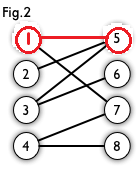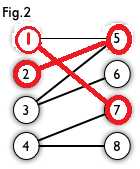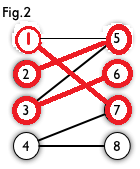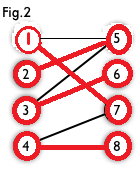# KM算法：

https://www.cnblogs.com/wenruo/p/5264235.html

https://www.cnblogs.com/logosG/p/logos.html?tdsourcetag=s_pcqq_aiomsg

### 代码：

#include<algorithm>
#include<iostream>
#include<limits.h>
#include<cstdlib>
#include<cstring>
#include<cassert>
#include<string>
#include<cstdio>
#include<bitset>
#include<vector>
#include<cmath>
#include<ctime>
#include<stack>
#include<queue>
#include<deque>
#include<list>
#include<set>
#define INT 9654234
#define mod 1000000007
typedef long long ll;
using namespace std;
const int MAXN = 305;
int N;
int ex_gir[MAXN];//每个妹子的期望值
int ex_boy[MAXN];//每个男生的期望值
bool vis_gir[MAXN];//记录每一轮匹配过的女生
bool vis_boy[MAXN];//记录每一轮匹配过的男生    每进行新的一轮，都要重新初始化这两个数组
int match[MAXN];//match[i]代表和i男生匹配的女生的编号
int slack[MAXN];//slack[i]代表i男生如果要获得女生的芳心，至少需要增加的期待值
int love[MAXN][MAXN];//记录每个妹子和男生的好感度
bool dfs(int gir)//dfs函数求的是编号为gir的女孩能否匹配到男生，如果能，返回true，否则，返回false
{
vis_gir[gir]=true;//标记
for(int i=1;i<=N;i++)
{
if(vis_boy[i])//我们规定每次匹配对于某个男生只访问一遍，如果先前访问过了，就换个男生
continue ;
int gap=ex_gir[gir]+ex_boy[i]-love[gir][i];
if(gap==0)//如果这个条件满足，说明编号为gir女孩和编号为i的男孩可能能够匹配成功
{
vis_boy[i]=true;//标记
if(match[i]==-1||dfs(match[i]))//如果这两个条件满足其中一个，说明编号为gir女孩和编号为i的男孩匹配成功
{
match[i]=gir;
return true;
}
}
else
slack[i]=min(slack[i],gap);//如果gap不等于0，说明当前状态编号为gir女孩和编号为i的男孩不可能匹配成功，更新slack[i]。
}
return false;
}
int km()
{
memset(match,-1,sizeof(match));
memset(ex_boy,0,sizeof(ex_boy));
for(int i=1;i<=N;i++)
for(int j=1;j<=N;j++)
ex_gir[i]=max(love[i][j],ex_gir[i]);//初始化ex_gir数组
for(int i=1;i<=N;i++)
{
fill(slack,slack+N+1,INT);
while (1)//这个while循环结束的条件是直到让编号为i的女生找到可以匹配的男生后
{
memset(vis_gir,false,sizeof(vis_gir));
memset(vis_boy,false,sizeof(vis_gir));
if(dfs(i))//如果这个条件满足，说明编号为i的女生找到了匹配的男生，换下一个女生,如果这个条件不满足，说明这个女生没有匹配到男生，让这个女生降低期望值后继续匹配
break ;
int t=INT;
for(int j=1;j<=N;j++)//寻找在这一轮匹配中没有匹配到的男生如果要获得女生芳心所需要增加的期待值的最小值
if(!vis_boy[j])
t=min(t,slack[j]);
for(int i=1;i<=N;i++)//让在这一轮匹配中匹配过的女生的期待值减小，匹配过的男生的期待值增加
{
if(vis_gir[i])
ex_gir[i]-=t;
if(vis_boy[i])
ex_boy[i]+=t;
else
slack[i]-=t;//因为有些女生的期待值减小了，所以这一轮没有被匹配过的男生得到女生的芳心所需要增加的期待值就变小了，所以slack数组中的相应的值要变小
}
}
}
int res=0;//计算好感和
for(int i=1;i<=N;i++)
res+=love[match[i]][i];
return res;
}
int main()
{
cin>>N;
for(int i=1;i<=N;i++)
for(int j=1;j<=N;j++)
cin>>love[i][j];
cout<<km()<<endl;
}

# Gale-Shapley---婚姻匹配算法算法

### 参考文献：

https://blog.csdn.net/Air_hjj/article/details/70828937

https://blog.csdn.net/lc_miao/article/details/78116064

### 代码：

#include<algorithm>
#include<iostream>
#include<limits.h>
#include <sstream>
#include<cstdlib>
#include<cstring>
#include<cassert>
#include<string>
#include<cstdio>
#include<bitset>
#include<vector>
#include<cmath>
#include<ctime>
#include<stack>
#include<queue>
#include<deque>
#include<list>
#include<set>
#define mod 1000000007
#define MAXN 1000
typedef long long ll;
using namespace std;
int ManArray[MAXN][MAXN],GirArray[MAXN][MAXN];//ManArray[i][j]代表编号为i的男生的第j位心仪女生是几号,GirArray[i][j]代表编号为i的女生的第j位心仪男生是几号
int Man[MAXN],Gir[MAXN];//Man[i]代表i号男生所匹配到的女生是几号，Gir[i]代表i号女生所匹配到的男生是几号
int ManStarPos[MAXN];//ManStarPos[i]代表i号男生现在匹配到的女生是他心目中的第几号心仪女生
int n;
stack < int >q;//始终存放没有匹配成功的男生的编号
int GetPositionFromLaday(int GirI,int ManI)//这个函数求的是编号为ManI的男生在编号为GirI的女生的心中的排名顺序
{
for(int i=0;i<n;i++)
if(GirArray[GirI][i]==ManI)
return i;//返回这个顺序
return -1;
}
void ManLookGir(int ManI)//为编号为ManI的男生匹配女生
{
int NowGir=ManArray[ManI][ManStarPos[ManI]];//得到这个男生应该匹配的女生的编号
if(Gir[NowGir]==-1)//如果这个条件满足，说明这个女生没有和她对应匹配的男生，那么就匹配上
{
Man[ManI]=NowGir;
Gir[NowGir]=ManI;
}
else//这个女生现在已经有男朋友了
{
if(OldMan<NowMan)//如果这个条件满足，说明这个女孩的现男友在这个女孩心中的排名要高于我们要匹配的这个男生的排名，这个女孩不换男朋友
{
ManStarPos[ManI]++;
q.push(ManI);
}
else//这个女孩更喜欢我们要匹配的这个男生，换男朋友
{
ManStarPos[Gir[NowGir]]++;//这是一个优化
q.push(Gir[NowGir]);
Man[ManI]=NowGir;
Gir[NowGir]=ManI;
}
}
}
int main()
{
cin>>n;
memset(Man,-1,sizeof(Man));//初始化这三个数组
memset(Gir,-1,sizeof(Gir));
memset(ManStarPos,0,sizeof(ManStarPos));
for(int i=0;i<n;i++)//输入每个男生心目中对女生的排序
for(int j=0;j<n;j++)
cin>>ManArray[i][j];
for(int i=0;i<n;i++)//输入每个女生心目中对男生的排序
for(int j=0;j<n;j++)
cin>>GirArray[i][j];
for(int i=0;i<n;i++)//刚开始对每个男生都进行一次匹配，从每个男生最心仪的女生开始匹配。如果从程序这个地方进入ManLookGir函数，那么每个男生都会和自己最心仪的女生进行一次匹配，因为是ManStarPos数组决定的，至于能否匹配成功，这就不一定了
ManLookGir(i);
while(!q.empty())
{
int i=q.top();
q.pop();
ManLookGir(i);
}
for(int i=0;i<n;i++)
cout<<"Man NO.: "<<i<<" Laday NO.: "<<Man[i]<<endl;
}

# 二分图例题：

https://wenku.baidu.com/view/63c1a01655270722192ef7c3.html

### 例题一：【HDU-1083】

http://acm.hdu.edu.cn/showproblem.php?pid=1083

### 代码：

#include<algorithm>
#include<iostream>
#include<limits.h>
#include <sstream>
#include<cstdlib>
#include<cstring>
#include<cassert>
#include<string>
#include<cstdio>
#include<bitset>
#include<vector>
#include<cmath>
#include<ctime>
#include<stack>
#include<queue>
#include<deque>
#include<list>
#include<set>
#define mod 1000000007
#define MAXN 400
typedef long long ll;
using namespace std;
int t;
int x,y;
int LinJie[MAXN][MAXN];
int nx[MAXN],ny[MAXN];
int visited[MAXN];
bool point(int u)
{
for(int v=1;v<=y;v++)
{
if(LinJie[u][v]&&!visited[v])
{
visited[v]=1;
if(ny[v]==-1||point(ny[v]))
{
nx[u]=v;
ny[v]=u;
return 1;
}
}
}
return 0;
}
int main()
{
cin>>t;
while(t--)
{
memset(LinJie,0,sizeof(LinJie));
memset(nx,-1,sizeof(nx));
memset(ny,-1,sizeof(ny));
cin>>x>>y;
for(int i=1;i<=x;i++)
{
int v,z;
cin>>v;
for(int j=0;j<v;j++)
{
cin>>z;
LinJie[i][z]=1;
}
}
for(int i=1;i<=x;i++)
{
if(nx[i]==-1)
{
memset(visited,0,sizeof(visited));
point(i);
}
}
int c=0;
for(int i=1;i<=x;i++)
{
if(nx[i]==-1)
{
cout<<"NO"<<endl;
c=1;
break;
}
}
if(!c)
cout<<"YES"<<endl;
}
}

# 例题二：POJ【3020】

### 思路：

https://blog.csdn.net/lyy289065406/article/details/6647040

### 代码：

#include<algorithm>
#include<iostream>
#include<limits.h>
#include <sstream>
#include<cstdlib>
#include<cstring>
#include<cassert>
#include<string>
#include<cstdio>
#include<bitset>
#include<vector>
#include<cmath>
#include<ctime>
#include<stack>
#include<queue>
#include<deque>
#include<list>
#include<set>
#define mod 1000000007
#define MAXN 50
#define maxn 2500
typedef long long ll;
using namespace std;
int t,n,m;
int root[MAXN][MAXN];//root数组存的是题目给定的数据
int LinJie[maxn][maxn];//LinJie数组存的是拆点完成后得到的二分图的一些信息
int nx[maxn],ny[maxn];//匈牙利算法所用到的数组
int visited[maxn];
int zk={{1,0},{-1,0},{0,1},{0,-1}};//用于往上下左右扩展的数组
void GetL()//通过root数组得到LinJie数组
{
for(int i=1;i<=n;i++)
{
for(int j=1;j<=m;j++)
{
if(root[i][j])
{
for(int k=0;k<4;k++)
{
int z=root[i+zk[k]][j+zk[k]];
if(z)
LinJie[root[i][j]][z]=1;
}
}
}
}
}
int point(int u,int l)//匈牙利算法
{
for(int v=1;v<=l;v++)
{
if(LinJie[u][v]&&!visited[v])
{
visited[v]=1;
if(ny[v]==-1||point(ny[v],l))
{
nx[u]=v;
ny[v]=u;
return 1;
}
}
}
return 0;
}
int main()
{
cin>>t;
char op;
while (t--)
{
memset(root,0,sizeof(root));
cin>>n>>m;
int l=0;
for(int i=1;i<=n;i++)
{
for(int j=1;j<=m;j++)
{
cin>>op;
if(op=='o')
root[i][j]=0; //没有城市，编号为0
else
root[i][j]=++l; //有城市，编号为l，我们给所有城市都定义一个编号
}
}
memset(LinJie,0,sizeof(LinJie));
GetL();//拆点得到我们需要的二分图
memset(nx,-1,sizeof(nx));
memset(ny,-1,sizeof(ny));
int res=0;
for(int i=1;i<=l;i++)
{
if(nx[i]==-1)
{
memset(visited,0,sizeof(visited));
res+=point(i,l);
}
}
cout<<l-res/2<<endl;//公式：无向二分图的最小路径覆盖 = 顶点数 – 最大二分匹配数/2
}
}

# 例题三：【HDU1150】

### 代码：

#include<algorithm>
#include<iostream>
#include<limits.h>
#include <sstream>
#include<cstdlib>
#include<cstring>
#include<cassert>
#include<string>
#include<cstdio>
#include<bitset>
#include<vector>
#include<cmath>
#include<ctime>
#include<stack>
#include<queue>
#include<deque>
#include<list>
#include<set>
#define mod 1000000007
#define MAXN 110
typedef long long ll;
using namespace std;
int A,B,n;
int e[MAXN][MAXN];
int nx[MAXN],ny[MAXN];
int visited[MAXN];
int point(int u)//匈牙利算法
{
for(int v=1;v<=B;v++)
{
if(e[u][v]&&!visited[v])
{
visited[v]=1;
if(ny[v]==-1||point(ny[v]))
{
nx[u]=v;
ny[v]=u;
return 1;
}
}
}
return 0;
}
int main()
{
while (1)
{
memset(e,0,sizeof(e));
memset(nx,-1,sizeof(nx));
memset(ny,-1,sizeof(ny));
cin>>A>>B>>n;
if(A==0)
break ;
for(int i=0;i<n;i++)//将题目输入的信息转化到邻接矩阵中去
{
int j,a,b;
cin>>j>>a>>b;
e[a][b]=1;
}
int ret=0;
for(int i=1;i<=B;i++)//基本的匈牙利算法
{
if(nx[i]==-1)
{
memset(visited,0,sizeof(visited));
ret+=point(i);
}
}
cout<<ret<<endl;//输出结果
}
}

# 关于建图的一些注意事项：